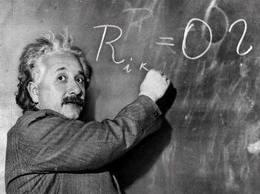Are You a Math Genius?

So...are you? Random math questions. No calculators Allowed! Have fun, rate, and follow! :)

published on June 09, 201448 responses 12 3.0★ / 5

A= p*r2
A=d*p
A=b*h
A=p*b*h
A=b*h*1/2

145 ways
780 ways
16 ways
720 ways
8 ways
6 ways

s=2
s=1
s=3
s=5

(5x+3)(x-5)
(5x-3)(x5)
(5x(x))(x-5)
(x-5)(x-5)